# 15.00 - ANSI Interval Expressions - Teradata Database

## Teradata Database SQL Functions, Operators, Expressions, and Predicates

Product
Release Number
15.00
Language
English (United States)
Last Update
2018-09-24

## ANSI Interval Expressions

### Purpose

Performs a computation on an Interval value (or value expression) and returns a single value of the same type.

### Definition

An interval expression is any expression that returns a result that is an INTERVAL value.

### interval_expression Syntax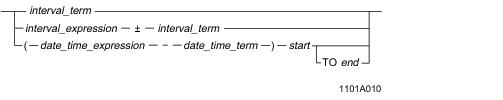### interval_term Syntax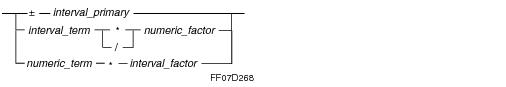### numeric_term Syntax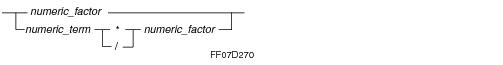### numeric_factor Syntax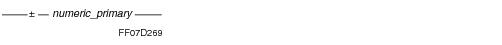where:

 Syntax element … Specifies … interval_expression an expression that evaluates to an INTERVAL value. The form of the expression is one of the following: a single interval_term the sum or difference of an interval_term and an interval_expression the difference between a date_time_expression and a date_time_term (enclosed by parentheses) preceding a start TO end phrase interval_term one of the following expressions: a single interval_factor an interval_term multiplied or divided by a numeric_factor the product of a numeric_term and an interval_factor interval_factor a signed interval_primary. date_time_expression an expression that evaluates to a DATE, TIME, or TIMESTAMP value. The form of the expression is one of the following: a single date_time_term the sum of an interval_expression and a date_time_term expression the sum or difference of a date_time_expression and an interval_term For more information on date_time_expression, see “ANSI DateTime Expressions” on page 826. date_time_term a single date_time_primary or a date_time_primary with a time zone specifier of AT LOCAL, AT [TIME ZONE] expression, or AT [TIME ZONE] time_zone_string. For more information on date_time_term, see “ANSI DateTime Expressions” on page 826. start a DateTime value with the following syntax that defines the beginning of a date or time interval: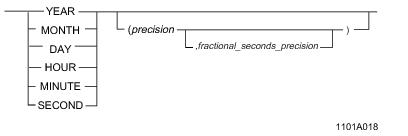where: precision specifies the permitted range of digits, ranging from one to four. The default precision is two. fractional_seconds_precision specifies the fractional precision for values of SECOND, ranging from zero to six. The default is six. MONTH and SECOND values are only permitted when used without TO end. TO end a DateTime value with the following syntax that defines the end of a date or time interval: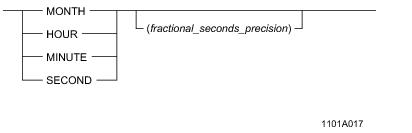where fractional_seconds_precision specifies the fractional precision for values of SECOND, ranging from zero to six. The default is six. The value for end must be less significant than the value for start. If start is a YEAR value, then end must be a MONTH value. numeric_factor a signed numeric_primary. numeric_term a numeric_factor or a numeric_term multiplied or divided by a numeric_factor. numeric_primary one of the following elements, any of which must have the appropriate numeric type: Column reference Numeric literal value Scalar function reference Aggregate function reference (table_expression) A scalar subquery. (numeric_expression) interval_primary one of the following elements, any of which must have the appropriate INTERVAL type: Column reference Interval literal value For details on Interval literals, see SQL Data Types and Literals. Scalar function reference Aggregate function reference (table_expression) A scalar subquery. (interval_expression)

### Examples of Interval Expression Components and Their Processing

The following examples illustrate the components of an interval expression and describe how those components are processed.

### Example of interval_term

The definition for interval_term can be expressed in four forms.

• interval_factor
• interval_term * numeric_factor
• interval_term / numeric_factor
• numeric_term * interval_factor
• This example uses the second definition.

`   SELECT (INTERVAL '3-07' YEAR TO MONTH) * 4;`

The interval_term in this operation is INTERVAL '3-07' YEAR TO MONTH.

The numeric_factor is 4.

The processing involves the following stages:

1 The interval is converted into 43 months as an INTEGER value.

2 The INTEGER value is multiplied by 4, giving the result 172 months.

3 The result is converted to '14-4'.

### Example of numeric_factor

This example uses a numeric_factor with an INTERVAL YEAR TO MONTH typed value.

`   SELECT INTERVAL '10-02' YEAR TO MONTH * 12/5;`

The numeric_factor in this operation is the integer 12.

The processing involves the following stages:

1 The interval is multiplied by 12, giving the result as an interval.

2 The interval result is divided by 5, giving '24-04'.

Note that very different results are obtained by using parentheses to change the order of evaluation as follows.

`   SELECT INTERVAL '10-02' YEAR TO MONTH * (12/5);`

The numeric_factor in this operation is (12/5).

The processing involves the following stages:

1 The numeric_factor is computed, giving the result 2.4, which is truncated to 2 because the value is an integer by default.

2 The interval is multiplied by 2, giving '20-04'.

### Example of interval_term / numeric_factor

The following example uses an interval_term value divided by a numeric_factor value.

`   SELECT INTERVAL '10-03' YEAR TO MONTH / 3;`

The interval_term is INTERVAL '10-03' YEAR TO MONTH.

The numeric_factor is 3.

The processing involves the following stages:

1 The interval value is decomposed into a value of months.

Ten years and three months evaluate to 123 months.

2 The interval total is divided by the numeric_factor 3, giving '3‑­05'.

The next example is similar to the first except that it shows how truncation is used in integer arithmetic.

`   SELECT INTERVAL '10-02' YEAR TO MONTH / 3;`

The interval_term is INTERVAL '10-02' YEAR TO MONTH.

The numeric_factor is 3.

The processing involves the following stages:

1 The interval value is decomposed into a value of months.

Ten years and two months evaluate to 122 months.

2 The interval total is divided by the numeric_factor 3, giving 40.67 months, which is truncated to 40 because the value is an integer.

3 The interval total is converted back to the appropriate format, giving INTERVAL '3-04'.

### Example of numeric_term * interval_primary

In this format, the value for numeric_term can include instances of multiplication and division.

`   SELECT 12/5 * INTERVAL '10-02' YEAR TO MONTH;`

The numeric_term is 12/5.

The interval_primary is INTERVAL '10-02' YEAR TO MONTH.

The processing involves the following stages:

1 The numeric_term 12/5 is evaluated, giving 2.4, which is truncated to 2 because the value is an integer by default.

2 The interval_primary is multiplied by 2, giving '20-04'.

### Example of numeric_term * ± interval_primary

This example multiplies a negative interval_primary by a numeric_term and adds the negative result to an interval_term.

`   SELECT (RACE_DURATION + (2 * INTERVAL -'30' DAY));`

The numeric_term in this case is the numeric_primary 2.

The interval_primary is INTERVAL -'30' DAY.

RACE_DURATION is an interval_term, with type INTERVAL DAY TO SECOND.

The processing involves the following stages:

1 The interval_primary is converted to an exact numeric, or 60 days.

2 The operations indicated in the arithmetic are performed on the operands (which are both numeric at this point), producing an exact numeric result having the appropriate scale and precision.

In this example, 60 days are subtracted from RACE_DURATION, which is an INTERVAL type of INTERVAL DAY TO SECOND.

3 The numeric result is converted back into the indicated INTERVAL type, DAY TO SECOND.

### Example of interval_expression

The definition for interval_expression can be expressed in three forms.

• interval_term
• interval_expression + interval_term
• (date_time_expression - date_time_term) start TO end
• This example uses the second definition.

`   SELECT (CAST(INTERVAL '125' MONTH AS INTERVAL YEAR(2) TO MONTH))    + INTERVAL '12' YEAR;`

The interval_expression is INTERVAL '125' MONTH.

The interval_term is INTERVAL '12' YEAR.

The processing involves the following stages:

1 The CAST function converts the interval_expression value of 125 months to 10 years and 5 months.

2 The interval_term amount of 12 years is added to the interval_expression amount, giving 22 years and 5 months.

3 The result is converted to the appropriate data type, which is INTERVAL YEAR(2) TO MONTH, giving '22-05'.

This example uses the third definition for interval_expression.

You must ensure that the values for date_time_expression and date_time_term are comparable.

`   SELECT (TIME '23:59:59.99' - CURRENT_TIME(2)) HOUR(2) TO SECOND(2);`

The date_time_expression is TIME '23:59:59.99'.

The date_term is the date_time_primary - CURRENT_TIME(2).

The processing involves the following stages:

1 Assume that the current system time is 18:35:37.83.

2 The HOUR(2) TO SECOND(2) time interval 18:35:37.83 is subtracted from the TIME value 23:59:59.99, giving the result '5:24:22.16'.

Here is another example that uses the third definition for interval_expression to find the difference in minutes between two TIMESTAMP values. First define a table:

`   CREATE TABLE BillDateTime`
`   (start_time TIMESTAMP(0)`
`   ,end_time TIMESTAMP(0));`

Now, determine the difference in minutes:

`   SELECT (end_time - start_time) MINUTE(4)`
`   FROM BillDateTime;`

The processing involves the following stages:

1 The start_time TIMESTAMP value is subtracted from the end_time TIMESTAMP value, giving an interval result.

2 The MINUTE(4) specifies an interval unit of minutes with a precision of four digits, which allows for a maximum of 9999 minutes, or approximately one week.

### Rules

The following rules apply to Interval expressions.

• Expressions involving intervals are evaluated by converting the operands to integers, evaluating the resulting arithmetic expression, and then converting the result back to the appropriate interval.
• The data type of both an interval_expression and an interval_primary is INTERVAL.
• An interval_expression must contain either year-month interval components or day-time interval components. Mixing of INTERVAL types is not permitted.
• Expressions involving intervals always evaluate to an interval, even if the expressions contain DateTime or Numeric expressions.
•
 IF an interval_expression contains … THEN the result … only one component of type INTERVAL is of the same INTERVAL type. a single DateTime value or a start TO end phrase contains the DateTime fields specified for the DateTime or start TO end phrase values. more than one component of type INTERVAL is of an INTERVAL type including all the DateTime fields of the INTERVAL types of the component fields.

### Normalization of Intervals with Multiple Fields

Because of the way the Parser normalizes multiple field INTERVAL values, the defined precision for an INTERVAL value may not be large enough to contain the value once it has been normalized.

For example, inserting a value of '99-12' into a column defined as INTERVAL YEAR(2) TO MONTH causes an overflow error because the Parser normalizes the value to '100-00'. When an attempt is made to insert that value into a column defined to have a 2-digit YEAR field, it fails because it is a 3-digit year.

Here is an example that returns an overflow error because it violates the permissible range values for the type.

First define the table.

`   CREATE TABLE BillDateTime`
`   (column_1 INTERVAL YEAR`
`   ,column_2 INTERVAL YEAR(1) TO MONTH`
`   ,column_3 INTERVAL YEAR(2) TO MONTH`
`   ,column_4 INTERVAL YEAR(3) TO MONTH );`

Now insert the value INTERVAL '999-12' YEAR TO MONTH using this INSERT statement.

`   INSERT BillDateTime (column_1, column_4) `
`   VALUES ( INTERVAL '40' YEAR, INTERVAL '999-12' YEAR TO MONTH );`

The result is an overflow error because the valid range for INTERVAL YEAR(3) TO MONTH values is -'999-11' to '999-11'.

You might expect the value '999-12' to work, but it fails because the Parser normalizes it to a value of '1000-00' YEAR TO MONTH. Because the value for year is then four digits, an overflow occurs and the operation fails.